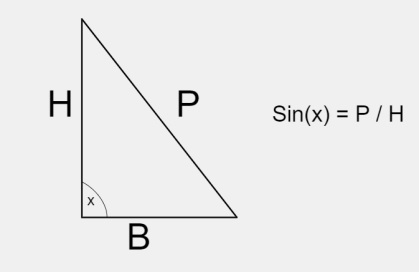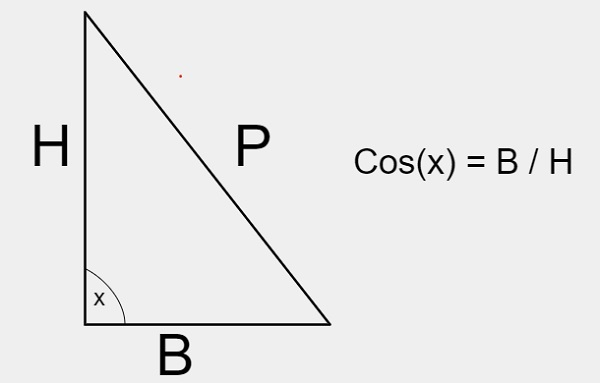# C++ Program to calculate the value of sin(x) and cos(x)

C++Server Side ProgrammingProgramming

Given with the input as angles and the task is to calculate the value of sin(x) and cos(x) corresponding to the given angle and display the result

### For Sin(x)

Sin(x) is a trigonometric function which is used to calculate the value of x angle.### Formula

$$\sin (x) = \displaystyle\sum\limits_{k=0}^\infty \frac{(-1)^{k}}{(2k+1)!}x^{2k+1}$$

### For Cos(x)

Cos(x) is a trigonometric function which is used to calculate the value of x angle.### Formula

$$\cos (x) = \displaystyle\sum\limits_{k=0}^\infty \frac{(-1)^{k}}{(2k)!}x^{2k}$$

Approach used in the below program is as follows

• Input the value of x angle for sin(x) and cos(x)
• Apply the formulas given for sin(x) and cos(x)
• Print the result

## ALGORITHM

START
Step 1-> declare function to calculate value of sin
void cal_sin(float n)
declare and set float acc = 0.0001, denominator, sinx, sinval
Set n = n * (3.142 / 180.0)
Declare float temp = n
Set sinx = n
Set sinval = sin(n)
Declare and set int i = 1
DO
set denominator = 2 * i * (2 * i + 1)
set temp = -temp * n * n / denominator
Set sinx = sinx + temp
Set i = i + 1
While(acc <= fabs(sinval - sinx))
print sinx
Step 2-> Declare function to calculate value of cos
void cal_cos(float n)
Declare and set float acc = 0.0001, temp, denominator, cosx, cosval
Set n = n * (3.142 / 180.0)
Set temp = 1
set cosx = temp
set cosval = cos(n)
Set int i = 1
Do
set denominator = 2 * i * (2 * i - 1)
Set temp = -temp * n * n / denominator
Set cosx = cosx + temp
Set i = i + 1
While(acc <= fabs(cosval - cosx))
print cosx
Step 3-> In main()
Declare float n = 30
Call cal_sin(n0
set n=60
Call cal_cos(n)
STOP

## Example

#include <iostream>
#include <math.h>
using namespace std;
//calculate value of sin
void cal_sin(float n) {
float acc = 0.0001, denominator, sinx, sinval;
n = n * (3.142 / 180.0);  //convert in radian
float temp = n;
sinx = n;
sinval = sin(n);
int i = 1;
do {
denominator = 2 * i * (2 * i + 1);
temp = -temp * n * n / denominator;
sinx = sinx + temp;
i = i + 1;
} while (acc <= fabs(sinval - sinx));
cout<<sinx;
}
//calculate value of cos
void cal_cos(float n) {
float acc = 0.0001, temp, denominator, cosx, cosval;
n = n * (3.142 / 180.0); //convert in radiam
temp = 1;
cosx = temp;
cosval = cos(n);
int i = 1;
do {
denominator = 2 * i * (2 * i - 1);
temp = -temp * n * n / denominator;
cosx = cosx + temp;
i = i + 1;
} while (acc <= fabs(cosval - cosx));
cout<< cosx;
}
int main() {
float n = 30;
cout<<"value of Sin is : "; cal_sin(n);
cout<<"\n";
n=60;
cout<<"value of Cos is : ";
cal_cos(n);
return 0;
}

## Output

value of Sin is : 0.500061
value of Cos is : 0.499847